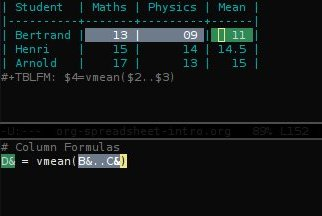## 序言

student maths physics
bertrand 13 09
henri 15 14
arnold 17 13

```| student  | maths | physics |
|----------+-------+---------|
| bertrand |    13 |      09 |
| henri    |    15 |      14 |
| arnold   |    17 |      13 |
```

student maths physics mean
bertrand 13 09 11
henri 15 14 14.5
arnold 17 13 15
means 15 12 13.5

<!– more –>

## 熟悉引用

```| student  | maths | physics | mean      |
|----------+-------+---------+-----------|
| bertrand |    13 |      09 | [formula] |
| henri    |    15 |      14 |           |
| arnold   |    17 |      13 |           |
```

## 第一个公式

`＃+TBLFM` 行包含表的所有公式，在手动编辑时应小心。

## 列公式和单元格公式

```| student  | maths | physics | mean |
|----------+-------+---------+------|
| bertrand |    13 |      09 |   11 |
| henri    |    15 |      14 |      |
| arnold   |    17 |      13 |      |
#+tblfm: @2\$4=vmean(\$2..\$3)
```

```| student  | maths | physics | mean |
|----------+-------+---------+------|
| bertrand |    13 |      09 |   11 |
| henri    |    15 |      14 | 14.5 |
| arnold   |    17 |      13 |   15 |
#+tblfm: \$4=vmean(\$2..\$3)
```

```| student  | maths | physics | mean |
|----------+-------+---------+------|
| bertrand |    13 |      09 |   11 |
| henri    |    15 |      14 | 14.5 |
| arnold   |    17 |      13 |   15 |
|----------+-------+---------+------|
| means    |    15 |      12 |      |
#+tblfm: \$4=vmean(\$2..\$3)::@5\$2=vmean(@2\$2..@4\$2)::@5\$3=vmean(@2\$3..@4\$3)
```

student maths physics mean
bertrand 13 09 11
henri 15 14 14.5
arnold 17 13 15
means 15 12

## 交互的编辑公式## Calc和Elisp公式

```Algebraic formulas use the operators `+', `-', `*', `/', and `^'. You
can use parentheses to make the order of evaluation clear. In the
absence of parentheses, `^' is evaluated first, then `*', then `/',
then finally `+' and `-'. For example, the expression

2 + 3*4*5 / 6*7^8 - 9

is equivalent to

2 + ((3*4*5) / (6*(7^8)) - 9
```

`Org` 表中，可使用引用而不是值来执行计算。

```#+CONSTANTS: pi=3.14159265358979323846
```

(不要忘了在 `#+CONSTANTS` 行上 键入 `C-c C-c` 以刷新 Local 设置)

```\$5='(substring (number-to-string \$pi) (round \$4) (1+ (round \$4)));N
```

Ahem. Let's parse this:

• `(substring S A B)`: 获取 `S` 字符串 `A``B` 之间的子串
• `(number-to-string \$pi)`: 把常量"Pi"转换成字符串
• `(round \$4)`: 获取 `\$4` 四舍五入后整数值
• `;N`: 把当前单元格的值当成整数，而不是字符串

## 调试公式

Student Maths Physics Mean Pi number
Bertrand 13 09 11 5
Henri 15 14 14.5 7
Arnold 17 13 15 9

```Substitution history of formula
Orig:   '(substring (number-to-string \$pi) (round \$4) (1+ (round \$4)));N
\$xyz->  '(substring (number-to-string 3.14159265358979323846) (round \$4) (1+ (round \$4)))
@r\$c->  '(substring (number-to-string 3.14159265358979323846) (round \$4) (1+ (round \$4)))
\$1->    '(substring (number-to-string 3.14159265358979323846) (round 11) (1+ (round 11)))
Result: 5
Format: NONE
Final:  5
```

## 很多, 还有更多

Last Updated 2017-06-01 Thu 13:40.
Render by hexo-renderer-org with Emacs 25.3.2 (Org mode 8.2.10)